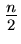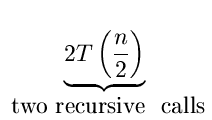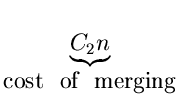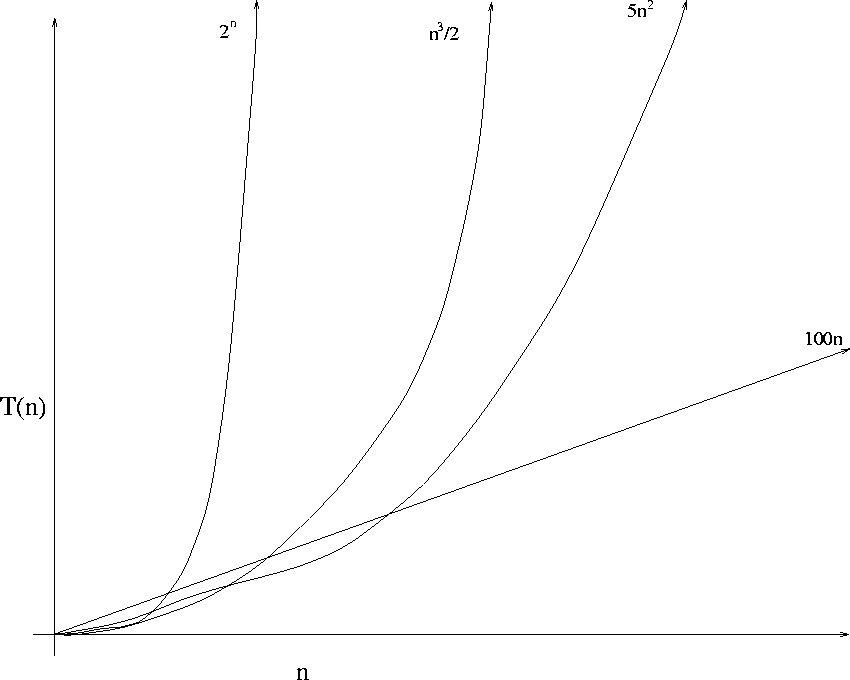Next:1.2.4 Role of the ConstantUp:1.2 Complexity of AlgorithmsPrevious:1.2.2 Examples

## 1.2.3 An Example: Complexity of Mergesort

Mergesort is a divide and conquer algorithm, as outlined below. Note that the function mergesort calls itself recursively. Let us try to determine the time complexity of this algorithm.

list mergesort (list L, int n);

{

if (n = = 1)

return (L)

else {

Split L into two halves L1 and L2 ;

return (merge (mergesort (L1), (mergesort (L2))

}

}

Let T(n) be the running time of Mergesort on an input list of size n. Then,

 T(n)C1  (if  n = 1)    (C1 is a constant)+(if  n > 1)

If n = 2k for some k, it can be shown that

T(n2kT(1) + C2k2k
That is, T(n) is O(nlog n).Table 1.1: Growth rate of functions

 Maximum Problem Size that can be solved in T(n) 100 1000 10000 Time Units Time Units Time Units 100 n 1 10 100 5 n2 5 14 45 n3/2 7 12 27 2n 8 10 13Next:1.2.4 Role of the ConstantUp:1.2 Complexity of AlgorithmsPrevious:1.2.2 Examples
eEL,CSA_Dept,IISc,Bangalore